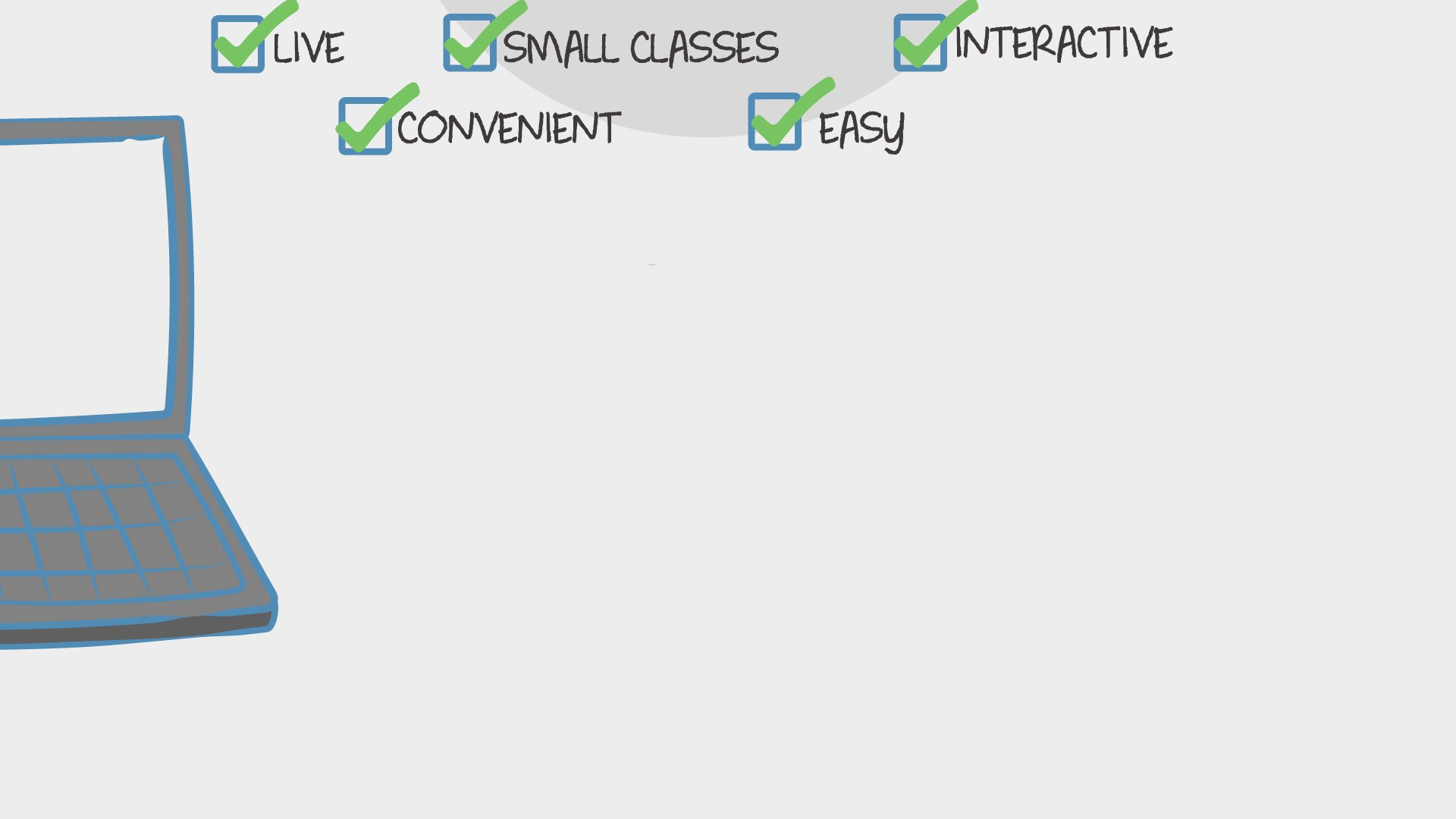Find Classes
Teach

Math

# 2nd Grade Multi-Day Math 3x/week

This on-going class focuses on using math in our every day lives.Taren Mangual
70 total reviews for this teacher
Completed by 1 learner
There are no upcoming classes.30 minutes
per class
3x per week
every week
6-10
year olds
1
learner per class
per learner - per class

### How does an "Ongoing" course work?

Meets on a weekly schedule, join any week, no need to catch up on previous material
Live video chats, recorded and monitored for safety and quality
Discussions via classroom forum and private messages with the teacher
Automatic payment every Sunday, cancel any time
Great for clubs and for practicing skills### How Outschool Works### There are no open spots for this class.

You can request another time or scroll down to find more classes like this.

## Description

### Class Experience

`This class is taught in English.`
```CCSS
2.G.A.3
Partition circles and rectangles into two, three, or four equal shares, describe the shares using the words halves, thirds, half of, a third of, etc., and describe the whole as two halves, three thirds, four fourths. Recognize that equal shares of identical wholes need not have the same shape.
CCSS
2.G.A.2
Partition a rectangle into rows and columns of same-size squares and count to find the total number of them.
CCSS
2.G.A.1
Recognize and draw shapes having specified attributes, such as a given number of angles or a given number of equal faces. Identify triangles, quadrilaterals, pentagons, hexagons, and cubes.
CCSS
2.OA.C.4
Use addition to find the total number of objects arranged in rectangular arrays with up to 5 rows and up to 5 columns; write an equation to express the total as a sum of equal addends.
CCSS
2.OA.C.3
Determine whether a group of objects (up to 20) has an odd or even number of members, e.g., by pairing objects or counting them by 2s; write an equation to express an even number as a sum of two equal addends.
CCSS
2.OA.B.2
Fluently add and subtract within 20 using mental strategies. By end of Grade 2, know from memory all sums of two one-digit numbers.
CCSS
2.OA.A.1
Use addition and subtraction within 100 to solve one- and two-step word problems involving situations of adding to, taking from, putting together, taking apart, and comparing, with unknowns in all positions, e.g., by using drawings and equations with a symbol for the unknown number to represent the problem.
CCSS
2.NBT.B.9
Explain why addition and subtraction strategies work, using place value and the properties of operations.
CCSS
2.NBT.B.8
Mentally add 10 or 100 to a given number 100–900, and mentally subtract 10 or 100 from a given number 100–900.
CCSS
2.NBT.B.7
Add and subtract within 1000, using concrete models or drawings and strategies based on place value, properties of operations, and/or the relationship between addition and subtraction; relate the strategy to a written method. Understand that in adding or subtracting three-digit numbers, one adds or subtracts hundreds and hundreds, tens and tens, ones and ones; and sometimes it is necessary to compose or decompose tens or hundreds.
CCSS
2.NBT.B.6
Add up to four two-digit numbers using strategies based on place value and properties of operations.
CCSS
2.NBT.B.5
Fluently add and subtract within 100 using strategies based on place value, properties of operations, and/or the relationship between addition and subtraction.
CCSS
2.NBT.A.4
Compare two three-digit numbers based on meanings of the hundreds, tens, and ones digits, using >, =, and < symbols to record the results of comparisons.
CCSS
2.NBT.A.1
Understand that the three digits of a three-digit number represent amounts of hundreds, tens, and ones; e.g., 706 equals 7 hundreds, 0 tens, and 6 ones. Understand the following as special cases:
CCSS
2.MD.D.10
Draw a picture graph and a bar graph (with single-unit scale) to represent a data set with up to four categories. Solve simple put-together, take-apart, and compare problems using information presented in a bar graph.
CCSS
2.MD.D.9
Generate measurement data by measuring lengths of several objects to the nearest whole unit, or by making repeated measurements of the same object. Show the measurements by making a line plot, where the horizontal scale is marked off in whole-number units.
CCSS
2.MD.C.8
Solve word problems involving dollar bills, quarters, dimes, nickels, and pennies, using \$ and ¢ symbols appropriately. Example: If you have 2 dimes and 3 pennies, how many cents do you have?
CCSS
2.MD.C.7
Tell and write time from analog and digital clocks to the nearest five minutes, using a.m. and p.m.
CCSS
2.MD.B.6
Represent whole numbers as lengths from 0 on a number line diagram with equally spaced points corresponding to the numbers 0, 1, 2,..., and represent whole-number sums and differences within 100 on a number line diagram.
CCSS
2.MD.B.5
Use addition and subtraction within 100 to solve word problems involving lengths that are given in the same units, e.g., by using drawings (such as drawings of rulers) and equations with a symbol for the unknown number to represent the problem.
CCSS
2.MD.A.4
Measure to determine how much longer one object is than another, expressing the length difference in terms of a standard length unit.
CCSS
2.MD.A.3
Estimate lengths using units of inches, feet, centimeters, and meters.
CCSS
2.MD.A.2
Measure the length of an object twice, using length units of different lengths for the two measurements; describe how the two measurements relate to the size of the unit chosen.
CCSS
2.MD.A.1
Measure the length of an object by selecting and using appropriate tools such as rulers, yardsticks, meter sticks, and measuring tapes.```
`1 hour 30 minutes per week in class, and maybe some time outside of class.`

## Teacher

```Since the beginning of my graduate studies in Educational Theatre at New York University I've held a strong interest in exposing others to creative ways of learning and providing a platform for self-expression.